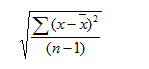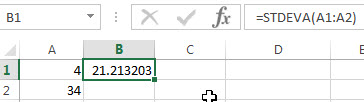# Excel STDEVA Function

This post will guide you how to use the STDEVA function with syntax and examples in Microsoft excel.

### Description

The Excel STDEVA function returns the standard deviation of a population based on a sample of numbers, text, and logical values. So the STDEVA function can evaluate the text and logical values that appear in cell references or arrays. The TEXT is evaluated as zero, TRUE is evaluated as 1, and FALSE is evaluated as zero. The standard deviation is used to identify the amount that a set of values differ from the average value. And if you want to calculate the standard deviation for an entire population, you can use the STDEVP or STDEV.P function.

The STDEVA function is a build-in function in Microsoft Excel and it is categorized as a Statistical Function.

The STDEVA function is available in Excel 2016, Excel 2013, Excel 2010, Excel 2007, Excel 2003, Excel XP, Excel 2000, Excel 2011 for Mac.

### Syntax

The syntax of the STDEVA function is as below:

`= STDEVA (value1, [value2], ..)`

Where the STDEVA function arguments are:

• Value1-This is a required argument.  The first number, text or logical value or cell reference in a sample of a population.
• Value2 -This is an optional argument.  Value arguments 2 to 255 corresponding to a sample of a population. And it can only accept up to 30 number arguments in Excel 2003 or older.

Note:

• Arguments can be the following: numbers; names, arrays, or references that contain numbers; text representations of numbers; or logical values, such as TRUE and FALSE, in a reference.
• Arguments that contain TRUE evaluate as 1; arguments that contain text or FALSE evaluate as 0 (zero).
• The STDEVA function is only used for its arguments are a sample of the population. and if the argument represents the entire population, you need to use the STDEVP function to calculate the standard deviation.
• Arguments that are error values or text that cannot be translated into numbers cause errors.
• If the argument contain the logical values and text representations of numbers and those values will be counted.
• STDEVA uses the following formula:### Excel STDEVA Function Examples

The below examples will show you how to use Excel STDEVA Function to calculate the standard deviation for data that represents a sample of the population.

1# to get standard deviation of a sample of numbers, using the following formula:

=STDEVA (A1:A2)### Related Functions

• Excel STDEV Function
The Excel STDEV function returns the standard deviation of a population based on a sample of numbers. This function has been replaced by the STDEV.S function in Excel 2010.The syntax of the STDEV function is as below:= STDEV (number1,[number2],…)…
• Excel STDEVP Function
The Excel STDEVP function returns the standard deviation of a population based on an entire population of numbers. This function has been replaced by the STDEV.P function in Excel 2010.The syntax of the STDEVP function is as below:= STDEVP(number1,[number2],…)…
• Excel STDEVPA Function
The Excel STDEVPA function returns the standard deviation of a population based on an entire population of numbers, text or logical values. The syntax of the STDEVPA function is as below:= STDEVPA (value1, [value2], …)…